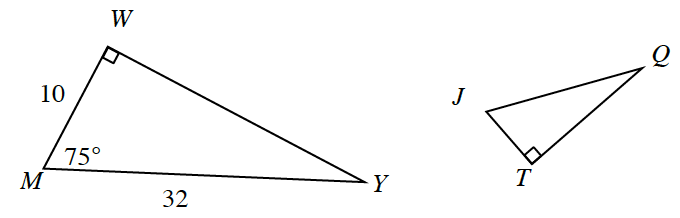### Home > INT1 > Chapter 10 > Lesson 10.1.1 > Problem10-20

10-20.

The two triangles below are congruent such that $ΔMWY ≅ ΔJTQ$. Make a sketch of the triangles on your paper and then label all three sides and all three angles. Note: The triangles are not drawn to scale.Pay attention to the order of the letters in the congruence statement.
Review the Math Notes box in section 7.1.4.
Since $ΔMWY$ is a right triangle, you can use the Pythagorean theorem to solve for side $\overline{WY}$.

Which side corresponds to $\overline{MW}$? $\overline{MY}$?